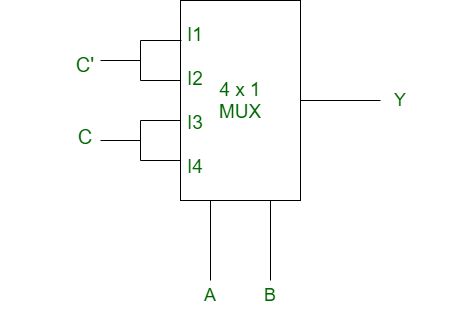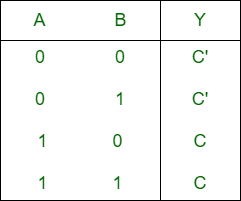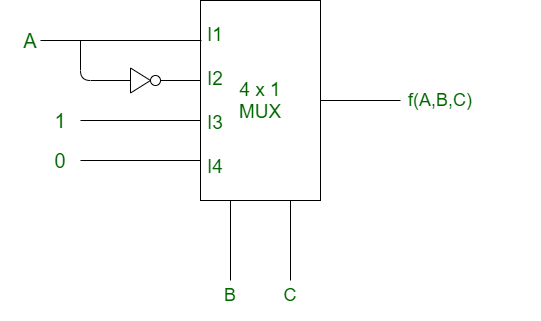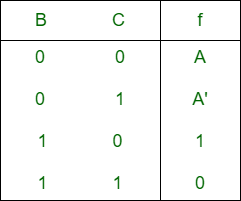# Solving multiplexer circuit

The procedure for solving and finding the output function of the given multiplexer is quite simple. Firstly we will discuss the procedure and then illustrate it with examples.

Procedure:

• Firstly truth table is constructed for the given multiplexer.
• Select lines in multiplexer are considered as input for the truth table.
• Output in truth table can be four forms i.e. ( 0, 1, Q, Q’).
• Now with the help of truth table we find the extended expression.
• Then the expression is minimized using boolean algebraic rules.
• Final function can be either in expression form or in SOP or POS form.

Example-1:
Given MUX is following,Explanation :

• Step-1: First draw the truth table. For the truth table, select lines A and B are the input.
According to the circuit,

```I0 = C' (hence first row of truth table will be C')
I1 = C'
I2 = C
I3 = C ```

I0, I1, I2, I3 are considered as output of 1st, 2nd, 3rd and 4th row of truth table respectively.• Step-2: Now we will find the expression of Y:
```Y = A'B'C' + A'BC' + AB'C + ABC
= A'C'(B' + B) + AC(B' + B)
= A'C' + AC
```

Example-2:
Given MUX,Explanation :

• Step-1: Truth table is following. For the truth table select lines B and C are input.
According to the circuit,

```I0 = A (hence first row of truth table will be A)
I1 = A'
I2 = 1
I3 = 0 ```

I0, I1, I2, I3 are considered as output of 1st, 2nd, 3rd and 4th row of truth table respectively.• Step-2: Now we will find the output:
```f(A, B, C) = AB'C' + A'B'C + BC'.1
= AB'C' + A'B'C + BC'(A + A')
= AB'C' + A'B'C + ABC' + A'BC'
=  100     001    110     010
= m(1, 2, 4, 6)```

Attention reader! Don’t stop learning now. Get hold of all the important CS Theory concepts for SDE interviews with the CS Theory Course at a student-friendly price and become industry ready.

My Personal Notes arrow_drop_upCheck out this Author's contributed articles.

If you like GeeksforGeeks and would like to contribute, you can also write an article using contribute.geeksforgeeks.org or mail your article to contribute@geeksforgeeks.org. See your article appearing on the GeeksforGeeks main page and help other Geeks.

Please Improve this article if you find anything incorrect by clicking on the "Improve Article" button below.